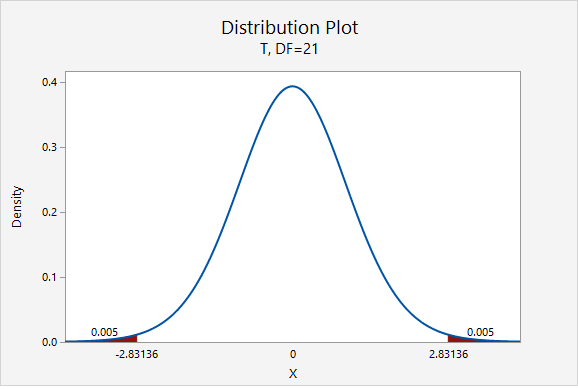# 8.2.2.1.2- Example: Sleep Deprivation

8.2.2.1.2- Example: Sleep Deprivation

In a class survey, students were asked how many hours they sleep per night. In the sample of 22 students, the mean was 5.77 hours with a standard deviation of 1.572 hours. That distribution was approximately normal. Let’s construct a 95% confidence interval for the mean number of hours slept per night in the population from which this sample was drawn.

This is what we know: $$n=22$$, $$\overline{x}=5.77$$, and $$s=1.572$$.

In order to compute the confidence interval for $$\mu$$ we will need the t multiplier and the standard error ($$\frac{s}{\sqrt{n}}$$).

$$df=n-1=22-1=21$$For a 95% confidence interval with 21 degrees of freedom, $$t^{*}=2.080$$

$$SE=\frac{s}{\sqrt{n}}=\frac{1.572}{\sqrt{22}}=0.335$$

Thus, our confidence interval for $$\mu$$ is: $$5.77\pm 2.080(0.335)=5.77\pm0.697=[5.073,\;6.467]$$

We are 95% confident that the population mean is between 5.073 and 6.467 hours.

What if we wanted to be more conservative and construct a 99% confidence interval?

The only thing that would change is our multiplier. Now, $$t^{*}=2.831$$.$$5.77\pm 2.831(0.335)=5.77\pm0.948=[4.822,\;6.718]$$

We are 99% confident that the population mean is between 4.822 and 6.718 hours.

  Link ↥ Has Tooltip/Popover Toggleable Visibility